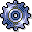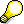Formula PlanningUse

Formula planning lets you use calculation dependencies to plan your cost center costs. You can define these dependencies as formulas in templates (see: Templates) that are independent of the cost center. In this way, cost centers with similar templates can use the same formulas.

Formula planning supports manual planning. It is particularly suitable for the planning of cost elements and activity inputs whose values are determined on many cost centers using similar logic. For example, Personnel Cost Planning, in which various cost elements (planned as statistical key figures) are derived from the number of employees.

For each cost center, you can create one planning template for activity-independent costs and key figures, and one planning template for activity-dependent costs and key figures. You can then store them in the cost center master record. You can also define sub-templates, to which reference can be made from the higher-level planning template.

In planning template maintenance, which you can find in Customizing, the following values can be used in the formula calculation:
For activity-independent planning templates: Total costs and total quantities
For activity-dependent planning templates: Fixed costs, fixed quantities and variable costs per activity unit, as well as variable quantities per activity unit.

You can use the following data from the SAP R/3 System in formula planning:

• All master data information for the cost center, for example, to define constraints.
• All master data information for the activity type, for example, to define constraints.
• Any primary planned costs
• Plan activity, scheduled activity and capacity for any cost center/activity type
• Any statistical key figures

You can also retain the cost centers, version, activity type and fiscal year as variable, so that the R/3 System can use the up-to-date evaluated cost center, etc. for the calculation.• The R/3 System calculates the costs in the planning template in the controlling area currency only.
• You cannot plan activities, revenues or order costs.

Prerequisites

During system configuration, you have created a planning template. (See also: The implementation guide (IMG) for Controlling, under Overhead Cost Controlling ® Cost Center Accounting ® Planning ® Formula Planning ® Maintain Template).

Features

Formula planning contains the following functions:

• Template maintenance

In planning template maintenance you define, per row, one or more formulas for an object (cost element, statistical key figure or calculation row) to calculate costs or quantities (see: Formulas). The R/3 System evaluates these formulas, during which it calculates one object for each row (cost element, statistical key figure). You can take Functions that allow the reading of plan and actual values, for example ACTIVITY_TYPE_ACTUAL_QTY or ACTIVITY_TYPE_PLAN_QTY and link these functions to basic formulas.

Using the interim results, which you can display in the Calculation Rows, the R/3 System can calculate complicated formulas. You can use activation (see: Activation) to represent conditions for which a formula is to be calculated.You maintain planning templates in Customizing.

When you evaluate a planning template for a cost center or a cost center group, the system uses the formulas defined in the template to calculate the costs or key figure values. To do this, it calculates each individual planning template for the given cost centers. If the object is a cost element or a statistical key figure, the system creates a plan record on the cost center for the cost element or statistical key figure. This plan record overwrites any manually planned record that may exist. It can be overwritten again in manual planning.

For the evaluation of planning templates, you can enter any cost centers cost center groups, or all cost centers. For these cost centers, the R/3 System selects the corresponding planning template and evaluates it. You can start the evaluation as often as required for one cost center.